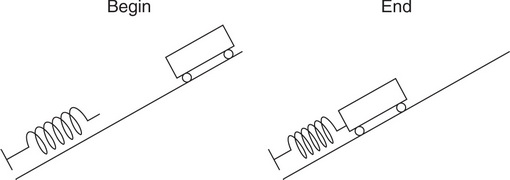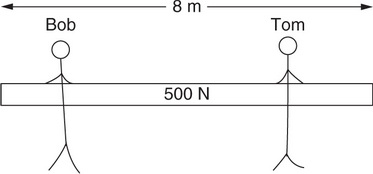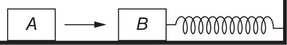# AP Physics 1 Practice Test 10

### Test Information10 questions18 minutes

1. The Space Shuttle orbits 300 km above Earth’s surface; Earth’s radius is 6,400 km. What is the gravitational acceleration experienced by the Space Shuttle?

2. A person stands on a scale in an elevator. He notices that the scale reading is less than his usual weight. Which of the following could possibly describe the motion of the elevator?

3. A textbook weighs 30 N at sea level. Earth’s radius is 6,400 km. Which of the following is the best estimate of the textbook’s weight on a mountain peak located 6,000 m above sea level?

4. A satellite orbits the moon in a circle of radius R. For the satellite to double its speed but maintain a circular orbit, what must the new radius of its orbit be?

5.A 0.5 kg cart begins at rest at the top of an incline, 0.06 m vertically above its end position. It is released and allowed to travel down the smooth incline, where it compresses a spring. Between the positions labeled "Begin" and "End" in the figure, the work done on the cart by the earth is 0.30 J; the work done on the cart by the spring is –0.20 J. What is the cart’s kinetic energy at the position labeled "End"?

6.Question below refers to the following information:

A rigid rod of length L and mass M sits at rest on an air table with negligible friction. A small blob of putty with a mass of m moves to the right on the same table, as shown in overhead view in the figure. The putty hits and sticks to the rod, a distance of 2L/3 from the top end.

How will the rod-putty system move after the collision?

7.Question below refers to the following information:

A rigid rod of length L and mass M sits at rest on an air table with negligible friction. A small blob of putty with a mass of m moves to the right on the same table, as shown in overhead view in the figure. The putty hits and sticks to the rod, a distance of 2L/3 from the top end.

What quantities, if any, must be conserved in this collision?

8.Bob and Tom hold a rod with a length of 8 m and weight of 500 N. Initially, Bob and Tom each hold the rod 2 m from the its ends, as shown in the figure. Next, Tom moves slowly toward the right edge of the rod, maintaining his hold. As Tom moves to the right, what happens to the torque about the rod’s midpoint exerted by each person?

9.An object of mass m hangs from two ropes at unequal angles, as shown in the figure. Which of the following makes correct comparisons between the horizontal and vertical components of the tension in each rope?

10.Question below refers to the following information:

Block B is at rest on a smooth tabletop. It is attached to a long spring, which in turn is anchored to the wall. Identical block A slides toward and collides with block B. Consider two collisions, each of which occupies a duration of about 0.10 s:

Collision I: Block A bounces back off of block B.

Collision II: Block A sticks to block B.

In which collision, if either, does block B move faster immediately after the collision?